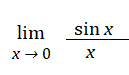# Indeterminate Limits & Expressions

Limit of Functions > Indeterminate Limits & Expressions

Contents:

## What is an Indeterminate Expression?

An indeterminate expression seems to have more than one answer, depending on how you evaluate it.

For example, the limitSeems to be zero if you use direct substitution: sin(0)/0. However, sin x and x are almost equal to each other as you get close to zero, which actually makes the limit 1.

This kind of conflict is why these expressions can’t be evaluated in many software programs; The system will balk if you try and enter one because it doesn’t know which way you want it evaluated. Wolfram’s The MATHEMATICA ® Book, Version 4 goes as far as calling indeterminate expressions “poison” for arithmetic computation.

## Indeterminate Expression Examples

Many of these expressions are found in mathematics:

• 00
• 0/0
• 0 x ∞
• ∞ – ∞
• ∞ / ∞
• infin;0
• 1

For example, 0 X ∞ might be 0 or ∞ (or any finite number in between). Any limit that results in an indeterminate expression when you substitute in x = a has to be evaluated carefully.

## How Do I Evaluate an Indeterminate Expression?

One workaround is to ignore the exact limit and evaluate the function close to a. In the sin(0)/0 example, you could evaluate the function at sin(0.01)/0.01 = 0.99998.

A more formal approach is L’Hospital’s Rule. The rule can only be used in special cases, but it makes some of these expressions fairly simple to evaluate. For example, all you need to do to evaluate sin(x)/x at x = 0 is:

1. Take the derivative of the top and bottom (treating each as functions). The derivative of sin(x) is cos(x) and the derivative of x is 1.
2. Make a new quotient with your answers from Step 1: cos(x)/x.
3. Find the limit of the new quotient. cos(0)/1 = 1

## Indeterminate limits

An “indeterminate” limit is one that can’t be found, at least not with the usual rules for finding limits. Indeterminate limits may not have limits at all, and if they do, they don’t indicate what those limits might be.

The most common indeterminate limits you’ll come across in calculus are {0/0} and {∞/∞}, but there are many others. Indeterminate limits include:

• Limits involving infinity: {∞ / ∞}, {∞ – ∞}.
• Exponential indeterminate forms:0, 00, 1
• Zeros types: {0/0} or {0 * ∞).

## How to Find Indeterminate Limits

Arguably, the easiest way to find these limits is to graph the function using a graphing calculator (or alternatively, look at the associated table of values). I used the free graphing calculator at Desmos.com to graph this function and find an approximate limit:

To find these limits using a more rigorous method, apply L’Hospital’s Rule.

## The Problem of Multiple Variables

The following function has the indeterminate limit 0/0:This particular function has several variables, so you can’t use L’Hospital’s rule to find the limit (Adillon, 2015).

To find a limit, you look at what happens when the function approaches a certain point x. You might not be able to find the limit at x exactly, but you can look at what happens from either side as the function approaches x; if both sides are equal, you’ve found the limit. If both sides aren’t equal, then the limit doesn’t exist. With a function with multiple variables, like the one above, the point can be approached by infinite different paths, making it practically impossible to hone in on a limit.

## References

Adillon, R. et al. (2015). Mathematics for Economics and Business. Edicions Universitat Barcelona. Retrieved May 21, 2019 from: https://books.google.com/books?id=HwNTDAAAQBAJ
Larson, R. & Edwards, B. (2017). Calculus of a Single Variable. Cengage Learning.
Neal, D. Indeterminate forms. Retrieved January 23, 2020 from: http://people.wku.edu/david.neal/136/Unit1/Indet.pdf
Wolfram, S. (1999). The MATHEMATICA ® Book, Version 4. Cambridge University Press.

CITE THIS AS:
Stephanie Glen. "Indeterminate Limits & Expressions" From StatisticsHowTo.com: Elementary Statistics for the rest of us! https://www.statisticshowto.com/indeterminate-limits/